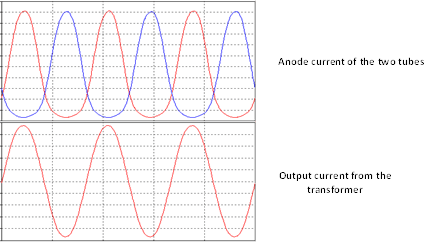Push-Pull amplifiers offer some advantages with respect to the single ended ones. Push-Pull transformers core can be made smaller than single ended ones and without air gaps. In fact, the current from the centre tap flows in opposite directions through the two half of the transformer and one current cancel the electro-magnetic effect of the other. Two additional advantages are the reduction of the harmonic distortions, and the increased output power, as discussed below.

When the vacuum tube’s operating range is situated in an asymmetrically nonlinear area, that is when the grid voltage lines intersect more densely the loadline on one side rather than the other, second order harmonics are generated. For instance, in Figure 21, grid voltage lines intersect the loadline more densely with an anode voltage higher than 400V, than with an anode voltage lower than 200V. Therefore, half signal is amplified very differently than the other half signal. Remember that the input signal corresponds to different grid voltages, that is different points, identified by the intersection of the grid voltage lines with the loadline. Figure 22 shows the effect of this nonlinearity. The upper plot shows the anode current of two vacuum tubes in push-pull. Since each vacuum tube amplifies a phase inverted signal, with respect to the other, the currents at the anodes of the two vacuum tubes are phase inverted as well. Note that the current signal is somehow flattened in the lower parts of the plots. This is because, as can be seen in Figure 21, there is less current variation on the right side of the loadline, compared to the left side of the loadline, in correspondence of the same grid voltage variation. This asymmetric behaviour introduces even order (mainly second order) harmonic distortions. However, given that the two signals are phase inverted, signal flattening occurs in one vacuum tube at time. When one vacuum tube is operating in a densier area, the other is operating in a less dense area. When one vacuum tube flattens the signal, the other vacuum tube does not. The two phase inverted signals are combined in the output transformer and even order harmonics, generated by the power vacuum tubes, are significantly attenuated, or cancelled, as can be seen in the lower plot of the figure. Note, however, that harmonic distortions introduced by previous stages or third order harmonics, introduced by symmetric nonlinearity, are amplified and not attenuated. Harmonic distortions produced by stages preceding the push-pull stage can be attenuated using global negative feedback, as will be discussed later in Section 4.4.Figure 22: Even order harmonic distortion in a push-pull amplifier.The upper plot shows the anode current of two vacuum tubes configured as push-pull when they amplify a signal. Note that when the amplified signal produced by one vacuum tube flattens in the lower part, due to the introduction of even order harmonics, the other vacuum tube compensates. The result is that the signal coming out from the output transformer, shown in the lower plot, is again an almost perfect sinusoidal signal, where second order harmonics are significantly reduced.

The push-pull configuration also offers the possibility of increasing the headroom, that is the operating range along the loadline. Larger headroom implies higher output power. When the amplitude of the input signal is too large, either the vacuum tube saturates or cuts-off (see Section 3.3). In a Single ended configuration, this is avoided by setting the bias so that it works in Class A condition (see Section 3.5) and taking care that the input signal is not too large, limiting therefore the amplifier headroom. However, in a push-pull amplifier we can set the bias so that vacuum tubes operate in class AB, with very low harmonic distortions. In class AB, one vacuum tube amplifies most of the signal and cuts-off when the input signal is below a certain threshold. Cut-off happens when the signal reaches the point where the loadline intersects the horizontal axis. When operating in class AB, given that the two vacuum tubes amplify phase inverted signals, if one vacuum tube cuts-off, the other vacuum tube still amplifies the inverted signal. This is depicted in the upper plot of Figure 23. The output transformer combines the two signals and an almost perfect signal is sent to the speaker, as shown in the lower plot of Figure 23. Operating in class AB allows setting the bias so that the vacuum tubes cuts-off alternatively, increasing the overall headroom.Figure 23: Push-pull amplifier operating in class AB.When operating in class AB, one vacuum tube amplifies just part of the whole signal, as shown in the upper plot. However, when one vacuum tube cuts-off the other still amplifies the signal, and vice versa. When the two signals are combined, in the output transformer, the original input signal is practically restored as shown in the lower plot.